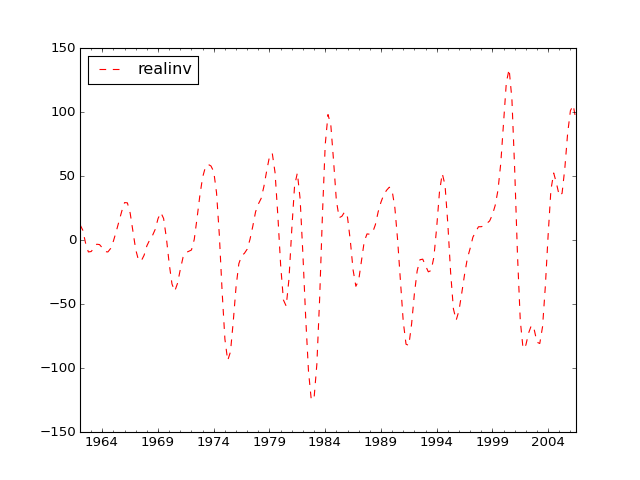# statsmodels.tsa.filters.bk_filter.bkfilter¶

`statsmodels.tsa.filters.bk_filter.``bkfilter`(X, low=6, high=32, K=12)[source]

Baxter-King bandpass filter

Parameters: X : array-like A 1 or 2d ndarray. If 2d, variables are assumed to be in columns. low : float Minimum period for oscillations, ie., Baxter and King suggest that the Burns-Mitchell U.S. business cycle has 6 for quarterly data and 1.5 for annual data. high : float Maximum period for oscillations BK suggest that the U.S. business cycle has 32 for quarterly data and 8 for annual data. K : int Lead-lag length of the filter. Baxter and King propose a truncation length of 12 for quarterly data and 3 for annual data. Y : array Cyclical component of X

Notes

Returns a centered weighted moving average of the original series. Where the weights a[j] are computed

```a[j] = b[j] + theta, for j = 0, +/-1, +/-2, ... +/- K
b = (omega_2 - omega_1)/pi
b[j] = 1/(pi*j)(sin(omega_2*j)-sin(omega_1*j), for j = +/-1, +/-2,...
```

and theta is a normalizing constant

```theta = -sum(b)/(2K+1)
```

References

Baxter, M. and R. G. King. “Measuring Business Cycles: Approximate
Band-Pass Filters for Economic Time Series.” Review of Economics and Statistics, 1999, 81(4), 575-593.

Examples

```>>> import statsmodels.api as sm
>>> import pandas as pd
```>>> cycles = sm.tsa.filters.bkfilter(dta[['realinv']], 6, 24, 12)
```>>> import matplotlib.pyplot as plt